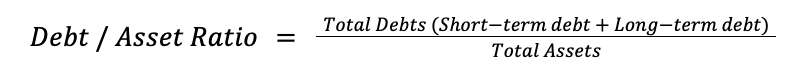Resources

Resources

Calculate Debt Ratio# Debt Asset Ratio Calculator

The debt to asset ratio calculator helps you easily calculate the debt to asset ratio. The metric shows the total amount of debts to the total amount of assets owned and shows the degree to which a company uses its debts to finance its assets.

You can also take a look at our other financial calculators, for example the debt to asset ratio (especially useful for companies) or the debt to income ratio (interesting for personal finance purposes).

## What is the debt-to-asset ratio?

The debt to asset ratio analyzes a company’s finances by combining short-term and long-term debt and dividing that value by the company’s total assets. The debt/asset ratio determines how much debt is used to finance an organization’s assets, and how those assets are utilized to service debt. In other words, the debt-to-asset ratio measures a company’s degree of leverage.

What’s considered a good debt to asset ratio?

Companies with a debt-to-asset ratio greater than 1 suggests that a company is funding a large part of their assets by debt. This means that the company has more liabilities than assets and has a significantly higher chance to not be able to pay off its debt.

Companies with a debt-to-asset ratio below 0.5 indicate that a larger part of the companies’ assets are funded by equity instead of debt. This allows companies more flexibility to use resources to invest in other opportunities and investments.

## Debt-to-asset ratio formula

The debt-to-asset ratio formula is calculated by dividing a company’s total debts by its total assets. As a formula, it’s expressed as:## How to calculate the debt-to-asset ratio

For this example, Company A has a total debt of \$33,450 and total assets of \$28,600. When the values are inputted into the debt-to-asset ratio formula it looks as follows:

Debt / Asset Ratio = \$33,450 / \$28,600 = 1.17

Company B, the next company in this example, has a total debt of \$21,460 and total assets of \$62,050. When the values are entered into the debt-to-asset ratio formula it looks as follows:

Debt / Asset Ratio = \$21,460 / \$62,050 = 0.35

Looking at these two companies in the example, it’s apparent that Company A’s debt-to-asset ratio is much higher than Company B’s debt-to-asset ratio. Being that Company A’s debt-to-asset ratio is greater than 1, it suggests that Company A is funding a large part of its assets strictly by debt. Having a high asset-to-debt ratio means that your company could be at risk of defaulting on its loans and debts.

On the other hand, Company B’s debt-to-asset ratio is much more favorable at 0.35. With a debt-to-asset ratio of less than 0.5, Company B has much more flexibility to invest in more assets and increase its overall revenue.

As observed from the example of Companies A and B, it’s more desirable for companies to have a lower debt-to-asset ratio so they aren’t relying too heavily on debt to finance their assets.## Matt here!

Your friends at EBizCharge have been building some great software that decreases days outstanding and lowers payment processing costs. You should check it out here.